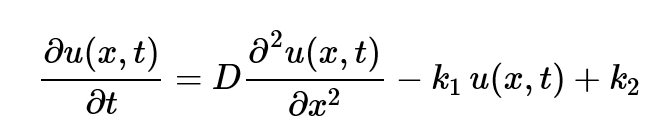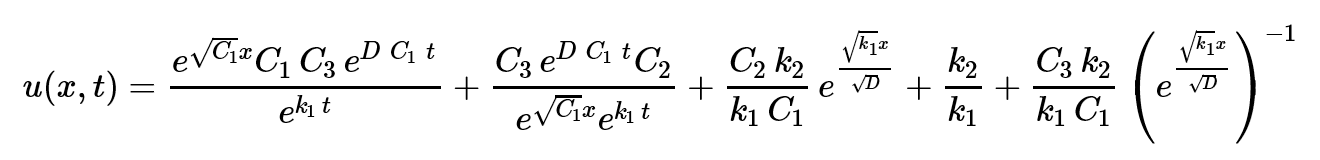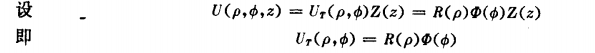• n个自变量的一阶线性偏微分方程的一般形式为 ∑j=1nbj∂u∂xj+cu=f(7) \sum_{j=1}^nb_j\frac{\partial u}{\partial x_j}+cu=f \tag{7} j=1∑n​bj​∂xj​∂u​+cu=f(7) 其中，bj=bj(x1,x2,...,xn),j=1,2,...,n,c=c...
n个自变量的一阶线性偏微分方程（$n\geq2$）
n个自变量的一阶线性偏微分方程的一般形式为
$\sum_{j=1}^nb_j\frac{\partial u}{\partial x_j}+cu=f \tag{7}$
其中，$b_j=b_j(x_1,x_2,...,x_n),j=1,2,...,n,c=c(x_1,x_2,..,x_n),f=f(x_1,x_2,...,x_n)$是已知的区域$D\subset \bold R^n$上的连续函数。
与$n-2$时一样，先来求解相应的齐次方程
$\sum_{j=1}^nb_j\frac{\partial \varphi}{\partial x_i}=0 \tag{8}$
为此，引入特征方程组
$\frac{ dx_1}{b_1}=\frac{dx_2}{b_2}=···=\frac{dx_n}{b_n} \tag{9}$
如选定变量$x_1,x_2,...,x_n$中的某一个（如$x_a$）作为自变量，特征方程（9）是n-1个一阶常微分方程$\frac{dx_j}{dx_n}=\frac{b_j}{b_n}(j=1,2,...,n-1)$组成的方程组，等价于一个n-1阶的常微分方程。它的解是n维空间中的曲线，称此积分曲线为一阶线性偏微分方程（7）的特征曲线。
如果引入参数t，特征方程组（9）可改写成
$\frac{dx_j}{dt}=b_j(x_1,x_2,...,x_n),\quad j=1,2,...,n$
积分曲线可表示为$\Gamma:\{x_j=x_j(t), \quad j=1,2,...,n\}$。如果能将特征方程组（9）中的某些方程凑成一个全微分形式的方程$d\varphi(x_1,x_2,...,x_n)=0$，则积分得到的关系式$\varphi(x_1,x_2,...,x_n)=h$（常数）称为常微分方程组（9）的一个首次积分。由常微分方程理论，一个n-1阶常微分方程存在且最多存在n-1个相互独立的首次积分。这n-1个独立的首次积分$\varphi_j(x_1,x_2,...,x_n)=h_j(j=1,2,...,n-1)$的联立即以隐函数的形式给出了（9）式的隐式通解（积分曲线族）。当n=2时，特征方程（9）只有一个独立的首次积分，即为积分曲线族。类似于n=2的情形，偏微分方程（8）的解与特征方程组（9）的首次积分之间有确定的关系。
定理：若$\varphi(x_1,x_2,...,x_n)=h$是特征方程组（9）在$D\subset \bold R^n$内的一个首次积分，则$\xi=\varphi(x_1,x_2,...,x_n)$是一阶线性偏微分方程（8）在D上的一个解。
证明：设$\varphi(x_1,x_2,...,x_n)=h$是特殊方程组（9）的一个首次积分，则沿着（9）式的任一条积分曲线$\Gamma:\{x_j=x_j(t),j=1,2,...,n\}$有
$d\varphi(x_1(t),x_2(t),...,x_n(t))=0$
即有
$\frac{\partial \varphi}{\partial x_1}\frac{dx_1}{dt}+\frac{\partial \varphi}{\partial x_2}\frac{dx_2}{dt}+···+ \frac{\partial \varphi}{\partial x_n}\frac{d x_n}{dt}=b_1\frac{\partial \varphi}{\partial x_1}+b_2\frac{\partial \varphi}{\partial x_2}+···+b_n\frac{\partial \varphi}{\partial x_n}=0$
由于过D内任一点有且仅有一条积分曲线，上式对于D内任一点成立，故$\xi=\varphi(x_1,x_2···,x_n)$是偏微分方程（8）在D上的解。
现在，求解一阶线性偏微分方程（7）。
由上述定理知，如果找到特征方程组（9）的n-1个独立的首次积分$\varphi_j(x_1,x_2,···,x_n)=h_j(j=1,2,···,n-1)$作自变量的变量代换
$\begin{cases} \xi_j=\varphi_j(x_1,x_2,···,x_n),\quad j=1,2,···,n-1 \\ \xi_n=\varphi_n(x_1,x_2,···,x_n) \end{cases} \tag{10}$
其中，$\varphi_n(x_1,x_2,···,x_n)$任取，使在D上
$J(\varphi_1,\varphi_2,···,\varphi_n)=\frac{\partial(\varphi_1,\varphi_2,···,\varphi_n)}{\partial(x_1,x_2,···,x_n)}= \begin{vmatrix} \frac{\partial \varphi_1}{\partial x_1} & \frac{\partial \varphi_2}{\partial x_2} & \cdots & \frac{\partial \varphi_n}{\partial x_n} \\ \frac{\partial \varphi_2}{\partial x_1} & \frac{\partial \varphi_2}{\partial x_2} & \cdots & \frac{\partial \varphi_n}{\partial x_2} \\ \vdots & \vdots & \ddots & \vdots \\ \frac{\partial \varphi_n}{\partial x_1} & \frac{\partial \varphi_n}{\partial x_2} & \cdots & \frac{\partial \varphi_n}{\partial x_n} \end{vmatrix} \neq 0$
代入（7），由链式法则得
$\sum_{j=1}^n b_j\frac{\partial u}{\partial x_j}=\sum_{j=1}^nb_j(\sum_{k=1}^n\frac{\partial \varphi_k}{\partial x_j}\frac{\partial u}{\partial \xi_k})=\sum_{k=1}^n(\sum_{j=1}^nb_j\frac{\partial \varphi_k}{\partial x_i})\frac{\partial u}{\partial \xi_k}$
由定理1知，当$k=1,2,···,n-1$时，有$\sum_{j=1}^nb_j\frac{\partial \varphi_k}{\partial x_j}=, u=u(x_1,···,x_n)$的方程（7）变成$u=u(\xi_1,\xi_2,···,\xi_n)$的方程
$(\sum_{j=1}^nb_j\frac{\partial \varphi_n}{\partial x_j})\frac{\partial u}{\partial \xi_n}+cu=f \tag{11}$
对$\xi_n$积分，可得（11）式的通解。再代会原来的自变量$(x_1,x_2,···,x_n)$，便得原方程（7）的通解。
特别地，当$c(x_1,x_2,···,x_n)=f(x_1,x_2,···,x_n)\equiv 0$时，方程（7）即为方程（8），变量代换（10）式后的新方程为
$\frac{\partial u}{\partial \xi_n}=0$
积分得通解
$u=g(\xi_1,\xi_2,···,\xi_{n-1})=g(\varphi_1(x_1,x_2,···,x_n),\varphi_2(x_1,x_2,···x_n),···,\varphi_{n-1}(x_1,x_2,···,x_n)),$
其中为任意$n-1$元$C^1$函数。
如果再给出未知函数在n维空间的一条曲线（非特征线）上的值，定出函数g，可得到特解。遗憾的是，一般而言，实际找出常微分方程的首次积分和确定函数关系g并非易事。
例1：求解初值问题
$\begin{cases} \sqrt{x}\frac{\partial u}{\partial x}+\sqrt{y}\frac{\partial u}{\partial y}+z\frac{\partial u}{\partial z}=0 \\ u|_{z=1}=xy \end{cases}$
解：特征方程为
$\frac{dx}{\sqrt x}=\frac{dy}{\sqrt u}=\frac{dz}{z}$
有两个独立的首次积分$\sqrt{x}-\sqrt{y}=c_1$和$2\sqrt{y}-lnz=c_2$，故齐次方程的通解为
$u=g(\sqrt x-\sqrt y,2\sqrt u-lnz)$
代入初始条件
$u|_{x=1}=g(\sqrt x-\sqrt y,2\sqrt y)=xy$
为了确定函数g，不妨令$p=\sqrt x-\sqrt y$和$q=2\sqrt y$，解得
$y=\frac{1}{4}q^2,\quad x=(p+\frac{1}{2}q)^2$
故
$g(p,q)=\frac{1}{4}q^2(p+\frac{1}{2}q)^2$
上述定解问题的解为
$u=(\sqrt y-\frac{1}{2}lnz)^2(\sqrt x-\frac{1}{2}lnz)^2$
例2：求解初值问题
$\begin{cases} \frac{\partial u}{\partial t}=x\frac{\partial u}{\partial x}+y\frac{\partial u}{\partial y}+u+xy \\ u|_{t=0}=\varphi(x,y) \end{cases}$
解：特征方程为
$\frac{dt}{1}=-\frac{dx}{x}=-\frac{dy}{y}$
积分得首次积分$xe^t=c_1,ye^t=c_2$。作变量代换
$\xi=xe^t,\quad \eta=ye^t, \quad \tau=t$
方程变为一阶常微分方程
$\frac{\partial u}{\partial \tau}=u(\xi,\eta,\tau)+\xi \eta e^{-2\tau}$
积分得通解
$u=e^{\tau}[\int \xi \eta e^{-2\tau}·e^{-\tau}d\tau+g(\xi,\eta)]\\ =-\frac{1}{3}\xi \eta^{-2\tau}+g(\xi,\eta)e^{\tau}=-\frac{1}{3}xy+g(xe^t,ye^t)e^t$
代入初始条件
$u|_{t=0}=-\frac{1}{3}xy+g(x,y)=\varphi(x,y) \\ g(x,y)=\frac{1}{3}xy+\varphi(x,y)$
得该定解问题的解
$u=-\frac{1}{3}xy+e^t[\frac{1}{3}xye^{2t}+\varphi(xe^t,ye^t)]$


展开全文• 对一种微分方程的求解，使用的是4阶龙格库塔方法，最后通过图形的形式给出方程的
• 精品文档 基础知识 偏微分方程的定问题 各种物理性质的定常即不随时间变化过程都可用椭圆型方程来描述其最典型最简单的形式是泊松 (Poisson) 方程 2 2 u u u 2 2 f (x , y) 1 x y 特别地当 f ( x, y) 0时即为...
• 在本文中，计算误差评估的根本问题是通过确定“等价”数学陈述来解决的，它是描述原始数学PDE陈述及其计算偏微分方程（PDE）。 换句话说，假设计算模型不能完全求解控制PDE，并且用于近似控制PDE的计算过程...
• 先将偏微分方程化成积分方程，再用逐步逼近法来确定方程的沃尔什级数形式的近似。本方法的特点是：①可以确定较高阶微分方程的近似，②沃尔什函数具有取值的简单性，从而简化了计算的编程工作。本文先将偏微分...
• 在假设不变子空间为二维的前提下，利用不变子空间方法有效构造了一个非线性偏微分方程组所允许的若干二维不变子空间，基于这些不变子空间获得方程组的一系列不同形式的广义变量分离；同时基于符号计算系统Maple给...
• 一般形式的二阶线性偏微分方程之间的共性和差异，往往可以从对这三类方程的研究中得到。 一维弦振动方程是双曲型的，一维热传导方程是抛物型的，二维拉普拉斯方程是椭圆型的。 以上三种方程描述的自然现象的...
弦振动方程、热传导方程与拉普拉斯方程。
这三类方程的形状很特殊，它们是二阶线性偏微分方程的三个典型代表。
一般形式的二阶线性偏微分方程之间的共性和差异，往往可以从对这三类方程的研究中得到。

一维弦振动方程是双曲型的，一维热传导方程是抛物型的，二维拉普拉斯方程是椭圆型的。

以上三种方程描述的自然现象的本质不同，其解的性质也各异。

这也从侧面说明了我们对二阶线性偏微分方程所进行的分类是有其深刻的原因的。

三类典型方程在数学性质上的差异往往是相应的物理现象的本质差异在数学上的表现。

下面我们以三类典型方程(波动方程、热传导方程和拉普拉斯方程)为例来叙述其差别。

对于一般的变系数方程，情况更复杂一些，但类似结论仍然成立。

解的光滑性

对于不同类型的方程来说，解的光滑性可以很不相同。对于弦振动方程来说，如果初始条件中高阶的导数不存在，那么解的高阶导数也就不存在；对于热传导方程，只要初始条件是有界的，那么其解是无穷可微的；对于拉普拉斯方程，它的解的光滑性更好，其解在定义域内都是解析函数。

解的极值性质

热传导方程和拉普拉斯方程都存在极值原理，但它们所采取的形式是有区别的。拉普拉斯方程解的极值只可能存在于边界。至于热传导方程，区域内部的最大值不能超过区域初始时刻和边界面上的最大值。双曲型方程通常不存在极值原理，这是因为波在叠加时可以出现扰动增大的情况。

影响区和依赖区

从影响区和依赖区来看，三类方程也有很大区别。波动方程的扰动是以有限速度传播的，因而其影响区和依赖区是锥体状的。对热传导方程而言，其扰动传播进行的十分迅速，某个点的其影响区是该点以上的整个上半平面，依赖区是整个初始值区间。拉普拉斯方程表示定常状态或平衡状态，因此不存在扰动传播的问题。

关于时间的反演

一物理状态的变化是否可逆，在数学上反映为所归结出来的方程关于时间变量是否是对称的，即以－t代替t后方程是否不变化。

拉普拉斯方程不存在此问题，双曲型方程是可逆的，热传导方程是不可逆的。

定解问题的提法

椭圆型方程：定解问题中只有边界条件而没有初始条件。故一般不提初边值问题和柯西问题。

抛物型方程：可以提初边值问题和柯西问题，其初始条件只需给出一个。

双曲型方程：可以提初边值问题和柯西问题，其初始条件需要给出两个。


展开全文• 这是在使用Julia解偏微分方程时相关的软件包的简要列表。 该信息主要从软件包存储库或已发布的报告或文章中收集。 如果 你以为错过了什么 您想修改或补充信息，或者 您希望不包含您的软件， 提出问题，甚至更好，使...
• Fourier变换在偏微分方程理论中占有相当重要的地位。本文通过经典的Fourier变换求解一维、二维热传导方程Cauchy问题的方法，推广Fourier变换到速降函数空间φ（Rn）和φ（Rn）上的线性连续泛函φ（Rn）广义函数空间...
• 常常看到有人抛出偏微分方程的问题, 明明求数值,一定要写成符号形式, 初边值条件也都写成符号形式, 比如 初值条件写成 u(x,0)=0;  边值条件写成 u(0,t)= f(t), u(L,t)=g(t) 然后, 求k1,k2 ...
 常常看到有人抛出偏微分方程的问题, 明明求数值解,一定要写成符号形式,
初边值条件也都写成符号形式, 比如初值条件写成 u(x,0)=0;
边值条件写成 u(0,t)= f(t), u(L,t)=g(t)

然后, 求k1,k2

偏微分方程问题很多数值求解都困难, 如果加上符号变量, 则必须精确解或解析解存在才可能解答;
从数值解到符号解,大大提高了难度, 可能让有解的问题变成无解;

采用不确定的函数作为边值条件更加容易导致问题.
这个问题初始的PDE是有符号解的;从而易知不是所有的初边值情况都会有解的, 必须满足上式方可;
所以, 平白把问题复杂化了;

看到这种提问的, 实在不想回答, 这是自己完全不通, 只想找个现成答案的伸手党的陋习;
今天的大学培养了数量巨大的大学生研究生,不少数理的基础并不扎实, 所以, 沦为伸手党. 这种弊病其实很容易改,

展开全文• 我们将问题简化为两次偏微分方程。 一个是系统演化的时间，另一个是“虚拟时间”，即类似于经典梯度下降迭代的连续迭代版本。 与纯数学的朋友交谈时，他们告诉我这个概念实际上是“叶子”，因此它更接近纯数学而不是...
• 求解偏微分的代码欧米茄方程 关于 Omega 方程是一个与时间无关的偏微分，它控制着水的垂直速度。 通常，控制流体的方程与时间无关，但一种特殊的近似，称为准地转近似，允许将复杂的 Navier-Stokes 方程简化为这种更...
• MATLAB要求微分方程能够表示成如下的一阶微分方程形式：  y’（t）= B * y（t） f（t） 其中，y 是一个状态向量，B是一个矩阵。应用前面一节讲的技术，将这个微分方程的MATLAB里面的定义成如下： xdot（2）...matlab
• 我们建议使用图神经网络来学习偏微分方程算子。 我们工作的关键创新在于，在精心设计的网络体系结构中，可以使用一组网络参数来描述无限维空间之间以及这些空间的不同有限维近似之间的映射。 多极图内核网络...
• 现在，可以利用诸如抽象数据类型（ADT）之类的新功能来开发KISS库，从而为微分方程的数值积分（如常微分方程和偏微分方程（ODE，PDE））提供令人敬畏的环境。 FOODIE专门针对PDE的半离散化产生的系统而设计，但不仅...
• De Giorgi迭代方法是处理椭圆型偏微分方程弱解性质的一种重要方法。运用De Giorgi的迭代技巧，讨论了一类散度形式椭圆型偏微分方程弱下的局部性质。
• 给出了非线性偏微分方程的表达式,运用此方法得到非线性强Klein-Gordon型方程的Kink、周期波等丰富精确.通过拟设法求得该方程的单重、双重及多重Compaction,给出了非线性色散强度、非线性耗散强度与非...
• 亥姆霍兹方程（英语：Helmholtz equation）是一个描述电磁波的椭圆偏微分方程，以德国物理学家亥姆霍兹的名字命名。其基本形式如下： 其中 ∇是哈密顿算子，k是波数，A是振幅。（来源：百度百科） 为将矢量亥姆霍兹...
圆柱坐标系统中亥姆霍兹方程的解
《微波与光电子学中的电磁理论》读书笔记1
亥姆霍兹方程：
亥姆霍兹方程（英语：Helmholtz equation）是一个描述电磁波的椭圆偏微分方程，以德国物理学家亥姆霍兹的名字命名。其基本形式如下：其中 ∇是哈密顿算子，k是波数，A是振幅。（来源：百度百科）
为将矢量亥姆霍兹方程化简为标量亥姆霍兹方程，这里采用了波格尼斯位函数法：（或许会在另一篇博客中专门写）
找到两个标量函数U(x),V(x), 使得E3只是U的函数，H3只是V的函数，各场分量与U，V有如下关系（此关系在本推导中未用到）：U和V满足二阶偏微分方程：$\nabla$2T为u1，u2的拉普拉斯运算：在柱坐标系统中，令u3为z，只讨论U的方程，用分离变量法，代入上面关于U的二阶偏微分方程，除以U，分离变量，得到圆柱坐标系统中，因此原式在这里成为式（1）：（分离变量）
代入式（1），得：因此有（v为分离常数，物理意义是角量子数？）：结合之前的Z的方程：解以上三个方程即可给出圆柱坐标中亥姆霍兹方程一般解：NB: 本人并不是光学专业，博文中如有哪部分理解阐释不到位，还希望各位积极斧正，和您的交流也是学习和巩固的过程。


展开全文• 通过观察与尝试,对的形态作预先假设,代入原方程,将一个难于求解的非线性偏微分方程化为一组易于求解的非线性代数方程,然后用待定系数法确定相应的常数,最后求得了含有各种形式微扰项的KdV方程的精确.
• Green公式的作用，到用Green函数解偏微分方程时候才能真正体现出来。 Green函数法解偏微分方程，具体就有很多应用了。 比如，简单的静电场的边值问题，直接就是Green函数模型提出的背景 比如，量子力学计算散射问题...Green
• 为给出非线性偏微分方程的更多精确类孤子,采用了投影Ricatti方程作为辅助方程,首先推导出了投影Ricatti方程的另外一种形式,证明这种特殊形式可以得到著名辅助方程φ~4方程的所有,研究结果表明,投影Ricatti...
• 通 过观察与尝试,对的形态作预先假设,代入原方程,将一个难于求解的非线性偏微分方程 化为一组易于求解的非线性代数方程,然后用待定系数法确定相应的常数,最后求得了含有 各种形式微扰项的 KdV方程的精确
• 利用Wronskian技巧构造了一类非线性孤子方程新的形式解．首先，给出非线性广义 Boussinesq方程的双线性形式，利用Wronskian技巧，构造出该非线性方程所满足的一个线性偏微分条件方程组．然后，求解该微分条件方程组...
• 关于非线性偏微分方程的单同宿轨道已有较好的研究结果，而双同宿轨道的研究因为其计算量大，形式复杂等原因并没有很好的结果.利用Hirota双线性算子方法，通过给出的相关变换，结合运用Maple软件，得到了非...
• 研究了SK-KP方程，该方程同时具有Sawada-Kotera方程和Kadomtsev-Petviashvili方程两个模型的双重特点，是一个可积性非常好的高阶非线性偏微分方程．利用F展开法与指数函数法相结合的方法，考察了该方程的精确，...
• 本文利用经典的李群分析方法讨论一类在工程和物理领域有着广泛应用的二维形式板振动的四阶偏微分方程的最优对称和精确．首先，利用无穷小生成元方法求得方程的不变群和最优对称，再对板方程进行化约降阶，从而获得...
• 通过将粒子速度场等效为势函数的梯度, 推导该速度场所满足的偏微分方程的弱形式; 应用Galerkin 有限元法和蒙特卡罗积分法, 推导出一个易于计算的弱形式常数近似. 仿真算例表明, 在一定初始条件下, 多峰型后验分布...
• 借助于符号计算软件Maple,通过一种构造非线性偏微分方程更一般形式行波的直接方法,即推广的Tanh-函数法,求解(2+1)维Burgers方程,得到该方程新的更一般形式的行波,包括扭状孤波,钟状,孤子和周期等,并对...
• 利用辅助函数法求解非线性耦合KdV方程组，把求解非线性偏微分方程组的问题转为求解代数方程组的问题，进一步应用Maple软件得到方程的十种精确行波，其中形式包括双曲函数、雅克比椭圆函数、三角函数和有理函数......